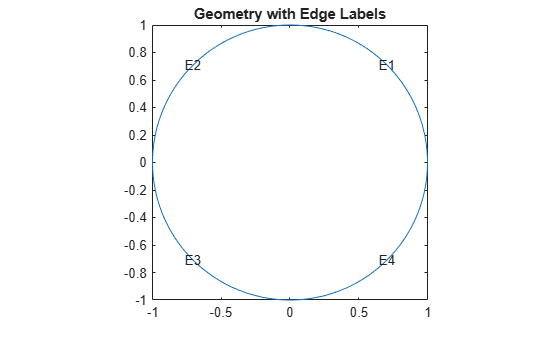# Minimal Surface Problem

This example shows how to solve the minimal surface equation

`$-\nabla \cdot \left(\frac{1}{\sqrt{1+{|\nabla u|}^{2}}}\nabla u\right)=0$`

on the unit disk $\Omega =\left\{\left(\mathit{x},\mathit{y}\right)|{\mathit{x}}^{2}+{\mathit{y}}^{2}\le 1\right\}$, with $u\left(x,y\right)={x}^{2}$ on the boundary $\partial \Omega$. An elliptic equation in the toolbox form is

`$-\nabla \cdot \left(c\nabla u\right)+au=f.$`

Therefore, for the minimal surface problem, the coefficients are as follows:

`$c=\frac{1}{\sqrt{1+{|\nabla u|}^{2}}},\phantom{\rule{1em}{0ex}}a=0,\phantom{\rule{1em}{0ex}}f=0.$`

Because the coefficient $c$ is a function of the solution $u$, the minimal surface problem is a nonlinear elliptic problem.

To solve the minimal surface problem using the programmatic workflow, first create a PDE model with a single dependent variable.

```numberOfPDE = 1; model = createpde(numberOfPDE);```

Create the geometry and include it in the model. The `circleg` function represents this geometry.

`geometryFromEdges(model,@circleg);`

Plot the geometry displaying the edge labels.

```pdegplot(model,'EdgeLabels','on'); axis equal title 'Geometry with Edge Labels';```Specify the coefficients.

```a = 0; f = 0; cCoef = @(region,state) 1./sqrt(1+state.ux.^2 + state.uy.^2); specifyCoefficients(model,'m',0,'d',0,'c',cCoef,'a',a,'f',f);```

Specify the boundary conditions using the function $u\left(x,y\right)={x}^{2}$.

```bcMatrix = @(region,~)region.x.^2; applyBoundaryCondition(model,'dirichlet',... 'Edge',1:model.Geometry.NumEdges,... 'u',bcMatrix);```

Generate a mesh.

```generateMesh(model,'Hmax',0.1); figure; pdemesh(model); axis equal```Solve the problem by using the `solvepde` function. Because the problem is nonlinear, `solvepde` invokes a nonlinear solver. Observe the solver progress by setting the `SolverOptions.ReportStatistics` property of the model to `'on'`.

```model.SolverOptions.ReportStatistics = 'on'; result = solvepde(model);```
```Iteration Residual Step size Jacobian: Full 0 1.8540e-02 1 2.8715e-04 1.0000000 2 1.2144e-06 1.0000000 ```
`u = result.NodalSolution;`

Plot the solution.

```figure; pdeplot(model,'XYData',u,'ZData',u); xlabel 'x' ylabel 'y' zlabel 'u(x,y)' title 'Minimal Surface'```## SupportGet trial now